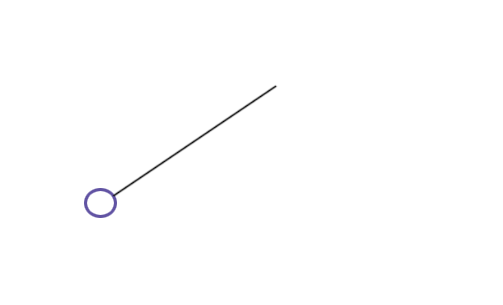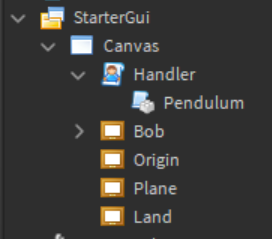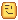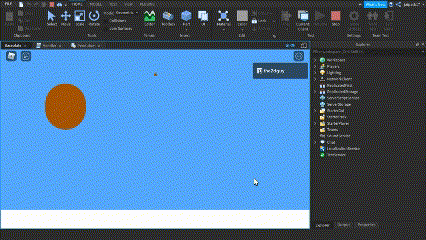# Gui Pendulum: Oscillatory Motion in a 2D Space

Recently, I started with Trigonometry and came across a fairly easy concept, I would like to share the same with you today. Lets talk about Oscillatory Motion.

Oscillatory motion is a type of periodic motion, the to and fro movement of an object. Take an example of the pendulum or a swing you see at a kid’s playground.The above gif gives you a brief visualization of what we are going to be making today. We’ll be scripting a Pendulum but on a 2D surface using Guis! I won’t be going indepth in the physics since there are so many forces acting on the object for example the force of gravity, atmospheric pressure and such, lets keep it simple today!

We’ll be using basic trigonometry in this tutorial, if you are unfamiliar with how trigonometry works, I highly recommend you to read the following article:

If you are equipped with your trigonometry knowledge, lets get going!

In the following picture, you can see a small little frame on the top and a big ball towards the bottom:

Be sure to set the Anchor Point of the bob to `(.5,.5)`

And my StarterGui looks something like this:Now that we have everything setup. In the above picture you can see a localscript and a ModuleScript inside of the localscript, named “Pendulum”. We’ll be using some OOP to code our pendulum, which is not necessary but lets use it for this tutorial! But before that, I’ll explain to you, how exactly we’ll be using trigonometry to get it to work.

In the figure above, we compare the bob’s initial position with the position after it moves. We have a few constants such as the origin, the length and the angle, the x and the y axis. If you look closely, they form a right triangle, so lets get our trigonometry out.

`sin(angle) = x/length` - sin(angle) is the opposite upon hypotenuse.

`cos(angle) = y/length` - cos(angle) is adjacent upon hypotenuse.

Using simple linear equations we can find out x and y:

`x = sin(angle) * length`
`y = cos(angle) * length`

`x = sin(angle) * length + origin.X`
`y = cos(angle) * length + origin.Y`

Gives us the location of the Bob. Now lets use this in our code.

``````local Pendulum = {}
Pendulum.__index = Pendulum

function Pendulum.new(origin, bob, length, theta, angularVelocity, angularAcceleration)
local self = setmetatable({
_origin = origin,
_bob = bob,
_length = length,
_theta = theta,
_vel = angularVelocity,
_acc = angularAcceleration
}, Pendulum)

return self
end

return Pendulum
``````

We have our basic code structure ready, in the above code, origin (vector2) is the point we saw in the above diagrams, bob (instance) is our small little ball that will move, length (number) is the distance between the origin and the bob and theta (number) is the angle between the intial position of bob and the final position of the bob. The other two parameters? Angular Velocity and Angular Acceleration? Yeah, lets keep that for the endNow lets apply our basic equations in our code:

``````function Pendulum:Oscillate()
local function simulate() -- helper function
local Bob = self._bob -- bob
local theta = self._theta -- angle
local length = self._length -- length
local origin = self._origin -- origin point

local x = length * math.sin(theta) + origin.X -- x abscissa
local y = length * math.cos(theta) + origin.Y -- y ordinate

Bob.Position = UDim2.new(0, x, 0, y) -- changing bob's position

self._theta = self._theta + .05 -- incrementing theta with a constant
end

game:GetService("RunService").RenderStepped:Connect(simulate) -- on renderstepped
end
``````

We used the same equations that we discussed about above, Now that we have our basic oscillation function ready, lets run the code!

In the “Handler” localscript:

``````local Pendulum = require(script.Pendulum)
local bob = script.Parent.Bob
local origin = script.Parent.Origin.AbsolutePosition

local newPendulum = Pendulum.new(origin, bob, 300, 0) -- origin position, bob, length of 300 pixels, 0 is the initial angle

newPendulum:Oscillate() -- starts the oscillation
``````

Hmmm, when we run the script, it doesn’t quite work yet, but you get the gist of how we are gonna make it work!Also you might have noticed, I did not make a “rope” for the pendulum, reason being: Rotating the rope requires another set of trigonometric formulas and 2D triangles so I’d rather not touch pivoting in this tutorial.

Lets understand what “angularVelocity” and “angularAcceleration” really means.

Velocity is a vector quantity thats the rate of change of the displacement of the object (bob).
Acceleration is the change in speed.

In a pendulum, the to and fro motion is caused because of these two quantities. There is a force applied in the opposite direction of the bob that makes it come back to its original position.

`acceleration of the pendulum = b * sin(theta)` where b is a constant.

And the angular velocity is the change of the angle.

Initially lets take angularAcc and angularVel to be 0. Using the formula of acceleration of the pendulum we discussed about above, we’ll simulate the oscillation:

``````function Pendulum:Oscillate()
local function simulate() -- helper function
local Bob = self._bob -- bob
local theta = self._theta -- angle
local length = self._length -- length
local origin = self._origin -- origin point

local x = length * math.sin(theta) + origin.X -- x abscissa
local y = length * math.cos(theta) + origin.Y -- y ordinate
Bob.Position = UDim2.new(0, x, 0, y) -- changing bob's position

self._acc = -0.01 * math.sin(theta) -- -0.01 is some constant, (equation above)
self._theta = self._theta + self._vel -- change in the angle using angularVelocity

self._vel = self._vel + self._acc -- velocity changes by acceleration
self._vel = self._vel * 0.99 -- damping
end

game:GetService("RunService").RenderStepped:Connect(simulate) -- on renderstepped
end
``````

You must have come accross a new term called “damping”, damping is basically us reducing energy of the oscillation, which will result in the bob coming to its initial point after its oscillation is over!

Lets test the code out:

``````local newPendulum = Pendulum.new(origin, bob, 300, 45, 0, 0) -- origin, bob, 300 length, 45 degree initial theta, 0 angular vel and angular acc

newPendulum:Oscillate()
``````

Amazing! You have your 2D pendulum ready, yeah the rope isn’t there, but lets keep pivot joints for some other day! After some time, you’ll notice the bob will slowly come to rest!

I hope you learned something new and had fun in this tutorial, hoping to see you all some other day in a new tutorial! Any questions? Ask me below or DM me!

Thats all from me today
Cheers!

Also you can reply below, if I missed something out in the tutorial, or something that needs to be added to the tutorial!

Feedback is also appreciated

### Placefile used for the tutorial: Pendulum - Tutorial.rbxl (30.0 KB)

17 Likes

Do you want a part 2 of this tutorial which will consist of other external forces and pressure, the rope using pivot rotations etc?

Would you like a Part 2 of this tutorial?
• Yes
• No

0 voters

I know it seems really bloated but i think implementing a rasterizer could make this process a lot easier. It doest necessarily need to be 3d btw. Simply only project the 2d coordinates. Brings the question though what are you suppose to do when they overlap when its in 2d.

Probably not since roblox really can’t keep up with rendering so many frames, better move the vector.

Can you elaborate a bit?

So I have made my own rasterizer and I can get 60fps when using simple geometry (less than 1000 triangles. Given I can use vertex normals to make a low res icosphere look smooth with phong shading thats neet. I was saying what are you suppose to do if two 2d objects, say 2 quads overlap. What should the color be?

The Color should be darker in shade considering two objects are overlapping.

Well then ig your depth buffer could be a integer, if a vertex position is interpolated check if that pixel’s depth buffers value and add to it. Then shade that pixel darker based on how high the number is. More over though, that sounds like transparency but thats the case if your objects are the same color

1 Like

That is a cool solution, but It may cause performance issues on many devices, moreover i feel like simply working with vectors and moving the gui object is an easier approach rather than using rasterizers.Well you could always just use bresenham to connect those thingies if yous like

2 Likes

## Changelog

• Updated the placefile.
• Added rope to the Pendulum. (Source code in the Placefile)

2 Likes

Uhhh, math to english translation?
just kidding, cool!

1 Like